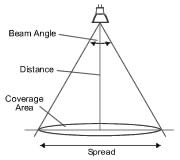# Lumen to Lux, MCD to Lumen, Light Spread Area Calculator

MilliCandelas(mcd) Lumen(lum) Converter

MCD (milicandela) to LUMEN

mcd (millicandela) to Lumens Converter This calculator is useful for calculating the luminous flux for LEDS where the angle is known. 1 candela of luminous intensity equals 1 lumen of luminous flux per steradian of solid angle, where a steradian respresents a cone whose surface area at the end equals the length of the cone squared. where: Luminous intensity is measured in Candela (cd) Luminous flux in measured in Lumens (lm), and the solid angle of the beam is measured in Steradian (sr). To use the calculator, enter any two known values and press "Calculate" to solve for the others.This Realtime Interactive Calculator
Converts millicandelas into lumens
 MilliCandelas(mcd): View Angle(deg): Lumens:

 Lumens-Lux Calculator

Here you can convert a lumens value from a product to a lux value if you know the beam angle and measurement distance. Enter the distance and beam angle, then either enter a Lumens value and hit 'Convert to Lux' or a Lux value and click 'Convert to Lumens'.

Lumens-Lux - Convert between Lumens and Lux
 Beam (view) Angle: degrees Distance: meters Lumens: Lumens (Lm) Lux: lux

Illuminance (lux): Illuminance is a measurement of the light intensity at any point. The light intensity drops exponentially the further away you get from the source (distance).
Luminous Flux (Lm): The luminous flux is a measure of the total light output from a source. For example, a 1 candela light source will product 1 lumen per square meter at the distance of 1 meter.
Beam Angle: The beam angle is the angle of radiation for a light source. For example a beam angle on 30 degrees means the light has a shape of a cone and its borders form a 15 degree angle with the center axis. A change in the beam angle affects the luminous intensity (lux) of a light source but not the luminous flux (Lumens).

Light Spread - Calculate the spread of light on a flat surface

 Beam (view) Angle: degrees Distance: metersEnter the values and click 'Calculate'.

Spread (m): The spread is the width of the light when it hits the flat surface.
Coverage Area (m2): The coverage area is the size of the circular area that the light illuminates on the flat surface.